# Uniform Circular Motion

Uniform Circular Motion –

When a body (or an object) moves in a circular path, it is called circular motion.

A circular path can be considered to be made up of an indefinite number of tiny sides, and a body moving along such a circular path changes its direction of motion continuously.

Suppose an athlete is running along a square track. While running along the square track having our sides, the athlete has to change his direction of motion four times (at the four corners A, B, C, D).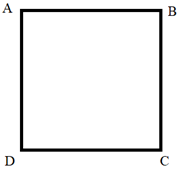Next, suppose the athlete runs along a hexagonal track. While running along the hexagonal track having six sides, the athlete has to change his direction of motion six times (at the six corners of the hexagonal track: A, B, C, D, E, F).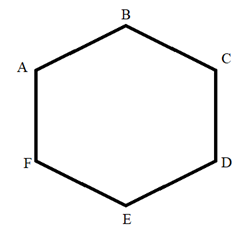Again, suppose the athlete now runs along an octagonal track. While running along the octagonal track having eight sides, the athlete has to change his direction of motion eight times (at the eight corners of the octagonal track: A, B, C, D, E, F, G, H).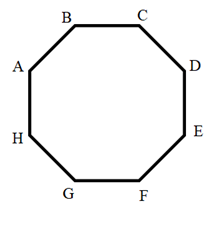Thus, as the number of sides of a track increases, the direction of an athlete running along it changes more and more frequently. Now, if the track has an indefinite number of tiny sides, then the shape of track becomes a circle or it becomes a circular track. And when the athlete runs along a circular track, then the direction of motion changes continuously.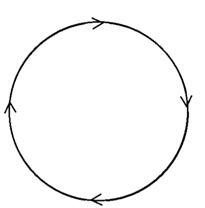So, if an athlete moves with a constant speed along a circular path, then the velocity of the athlete will not be constant because velocity is speed in a specified direction and here the direction of speed changes continuously.

Hence, the velocity of a body moving on a circular track changes continuously even if it is moving with constant speed because its direction of motion is changing continuously.

Now, we all know that acceleration refers to rate of change of velocity. Therefore, the motion of a body moving on a circular track is called accelerated motion since, its velocity changes continuously.

We will now define uniform circular motion. When the body moves in a circular path with uniform speed (constant speed), its motion is called uniform circular motion. It is possible for a body to move in a circular path with uniform speed as long as it is travelling equal distances in equal intervals of time. But the velocity of the body is not uniform due to continuous change in direction.

It is to be noted that a force is needed to produce circular motion. This can be understood by the example of a stone tied to a thread and rotated in a circular path. The pull of the thread is the force which makes the stone move in a circle and this pull is provided by the hand of the person holding the thread. The force which is needed to make an object travel in a circular path is called centripetal force. In the case of planets moving around the sun, the centripetal force is the gravitational pull of the sun and in case of satellites moving around the earth, the centripetal force is the force of gravity of earth.

Let us look at some of the examples of uniform circular motion –

1. Artificial satellites move in uniform circular motion around the earth: when an artificial satellite goes around the earth in a circular orbit with constant speed, its velocity is not constant because the direction of motion of satellite is changing continuously. Thus, the motion of satellite around the earth is accelerated. It is the force of gravity of earth which keeps the satellite in circular orbit around it.

2. The earth moves around the sun in uniform circular motion so, the motion of earth sround the sun is accelerated. It is the gravitational force of the sun which keeps the earth moving in a circular orbit around it.

3. An athlete (or a cyclist) moving on a circular track with a constant speed exhibits uniform circular motion. This motion is accelerated because of continuous change in direction of motion.

4. The tip of a second’s hand of a watch exhibits uniform circular motion on the circular dial of the watch. The speed of a second’s hand is constant but its velocity is not constant hence, its motion is accelerated.

Formula to calculate the speed of a body in uniform circular motion –

When a body takes one round of a circular path, then it travels a distance equal to its ‘circumference’ which is given by 2πr, where is the radius of the circular path.

The speed of a body is given by the formula :

v = 2πr/t

Where, v = speed

π = 22/7

r = radius of circular path

t = time taken for one round of circular path

Example –

A cyclist goes around a circular track once every 2 minutes. If the radius of the circular track is 105 metres, calculate his speed. (Given, π = 22/7)

Solution –

We know that for a body moving in a circular path

v = 2πr/t

Here, speed (v) =?

Time taken for one round (t) = 2 minutes = 2×60 seconds = 120 seconds

π = 22/7

Substituting these values in above equation, we get

v = (2×22×105)/(7×120)

v = 5.5 m/s

### NCERT Solutions for Class 9 Science Chapter 8 Motion – Uniform Circular Motion

NCERT textbook – Page 112 and 113

Question 1 –

An athlete completes one round of a circular track of diameter 200 m in 40 s. What will be the distance covered and the displacement at the end of 2 minutes 20 s ?

Solution –

Total time = 2 minutes 20 seconds

= 2×60 seconds + 20 seconds

= 140 seconds

Now, number of rounds completed in 40 seconds = 1

Number of rounds completed in 140 seconds = (1/40) × 140 = 3.5 rounds

Diameter of circular track = 200 m

Radius of circular track = 200/2 = 100 m

Distance covered in 1 round = circumference of circular track

= 2πr

= 2 × (22/7) × 100 m = 628.57 m

Distance covered in 3.5 rounds = (3.5 × 628.57) m

= 2200 m

Displacement after 3.5 rounds: after 3 rounds the athlete comes back to his starting point on the track and then further covers half of the total distance.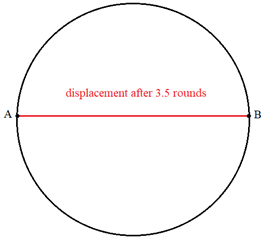So after 3.5 rounds, athlete comes at position B. The shortest distance between A and B is equal to the diameter of circular track i.e. 200m.

Conclusion – The motion of a body around a circular path is called circular motion. When a body performs circular motion with constant speed it is called uniform circular motion. The speed of a body in a uniform circular motion remains constant but the velocity changes continuously since, the direction of motion is changing continuously. Hence, uniform circular motion is an accelerated motion. The force required for circular motion is called centripetal force.The speed of a body performing uniform circular motion is given by the formula, v = 2πr/t where r is the radius of the circular track, t is the time required for completing one round of the circular track.